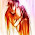Friday, June 4, 2010

Euler Tour

A famous drawing problem for kids, "You are given a picture, can you draw it without lifting up your pen?"... Well, in graph theory, we can determine this by checking the Euler Tour in the graph.Actually this is about Euler Circuits and Eluer Paths. We know the conditions for an undirected graph, and we can extend it for directed graphs as well.

Undirected Graph

An undirected graph will have Eulerian tour ( either path or circuit ) if the following conditions hold:
• The graph is connected.
• Each node has even degree, or exactly two nodes have an odd degree.

Directed Graph

A directed graph will have Eulerian tour ( either path or circuit ) if the following conditions hold:
• The undirected representation of the graph is connected.
• The difference between indegree and outdegree of each node is at most 1.
• Each node has equal indegree and outdegree, or, there is exactly two nodes which has different indegree and outdegree, and exactly one of them has indegree - outdegree = 1 and the other has outdegree - indegree = 1.

So, we can do this easily with the help of a bfs subroutine.
You might also like to read this and this.

1.Hello Mr. Hasan, can I ask you a doubt regarding bitwise operations via email?

Thank you.

2.Probably, YES :D

3.Can I have your email-id then?

4.5.